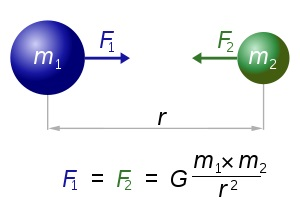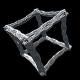# More adventures with 3D Gravity simulations and OpenCL

History

3D Gravity simulations are something I have been interested in for many years now. Some worked, some didn’t, some were more realistic than others.

The first 3D Gravity simulation movie I still have on my YouTube channel is this one from way back in May of 2007. Low res with only a bunch of blurry objects.

Since then I have increased the details and object counts. I also started experimenting with OpenCL for big speedups that allowed many more objects to be simulated in a reasonable time frame.

Moving forward to now

For this latest post I went back and rewrote my code and the OpenCL kernel code to correctly compare every object to all other objects in the gravity calculations. The simulation is using Newton’s law of universal gravitation.Every point mass attracts every single other point mass by a force acting along the line intersecting both points. The force is proportional to the product of the two masses and inversely proportional to the square of the distance between them.

How this simulation works

I am using software OpenGL on the CPU for all the rendering of the visuals and CPU and OpenCL for the gravity calculations. OpenCL code runs on your graphics card GPU and GPUs are great at running lots of small bits of code fast at the same time. The gravity formula maths is perfect for multi threading. Every objects velocity and acceleration can be calculated at the same time as the other objects.

The basics of using OpenCL is you fill arrays with the information you want the OpenCL code to use (for 3D gravity I am passing position, velocity, acceleration and mass of the objects), pass it to OpenCL, run the code on the GPU, and then read back the results from the GPU when it is done.

This is the current OpenCL code I am using for these latest simulations. Each of the arrays passed (posx, posy, etc) contain all the current objects, ie for a 1 million object simulation the posx array has 1,000,000 floating point values to cover every object’s X position in 3D space.

``````
__kernel void Gravity3DKernel( __global float * posx,
__global float * posy
__global float * posz,
__global float * velx,
__global float * vely,
__global float * velz,
__global float * accx,
__global float * accy,
__global float * accz,
__global float * mass,
__global float * mingravdist)
{
int index=get_global_id(0);
float dx,dy,dz,distance,force;
float positionx=posx[index];
float positiony=posy[index];
float positionz=posz[index];
float mingravdistsqr=mingravdist[index]*mingravdist[index];
float accelerationx=0;
float accelerationy=0;
float accelerationz=0;
float thismass=mass[index];
for(int a=0; a<get_local_size(0); a++) {
if (a!=index) {
dx=posx[a]-positionx;
dy=posy[a]-positiony;
dz=posz[a]-positionz;
distance=sqrt(dx*dx+dy*dy+dz*dz);
dx=dx/distance;
dy=dy/distance;
dz=dz/distance;
//old method - all objects are assumed to have the same mass
//force=1/(distance*distance+mingravdistsqr);
//new method - allows objects to have different masses
force=(thismass*mass[a])/(distance*distance+mingravdistsqr);
accelerationx+=dx*force;
accelerationy+=dy*force;
accelerationz+=dz*force;
}
}
velx[index]+=accelerationx;
vely[index]+=accelerationy;
velz[index]+=accelerationz;
accx[index]=accelerationx;
accy[index]=accelerationy;
accz[index]=accelerationz;
}```
```

The kernel code loops through every object and calculates the forces against every other object. This is the naive unoptimized O(n2) version of the algorithm. Once all the loops are finished the new object velocity and acceleration values are read back from the GPU memory into local memory and then the CPU can access the results. All of the object positions are then updated using the new velocity and acceleration values and then displayed. For displaying the objects I am using the old software only OpenGL billboard quads. A billboard quad is a texture on a quad (rectangle) that always faces the “camera” in OpenGL. If you put a nicely shaded and transparent “blob” as the texture it looks like a simple star and blends in with other stars.

Calculation Times

Using the above code allows me to process millions of objects in a reasonable time frame. For these simulations the slowest part is always the display and CPU calculations. The OpenCL is always the fastest part of the simulations.

Here are a few stats for how quick (slow) these simulations are on a PC with an i7 CPU and GTX 1080 GPU rendering 4K resolution frames;

250,000 objects – 856 ms per frame – 31 ms OpenCL time
500,000 objects – 1606 ms per frame – 57 ms OpenCL time
1,000,000 objects – 3124 ms per frame – 91 ms OpenCL time
5,000,000 objects – 15336 ms per frame – 430 ms OpenCL time
10,000,000 objects – 30638 ms per frame – 864 ms OpenCL time

The reason the per frame time is so much longer than the OpenCL is that I am still using software OpenGL to render all the particles. Software OpenGL falls back to the older v1.1 OpenGL DLLs provided by Microsoft in Windows and has no benefits of hardware acceleration. On my to do list is getting the OpenGL code up to date to use hardware acceleration, then the above times should come way down. Rendering the objects as pixels rather than billboard quads is roughly 1/3 the time, but the billboard quads give a much nicer blended display.

Results

Here is a new sample 4K resolution 3D Gravity movie.

After the last movie I went back and improved the color shading code and added the option for a “black hole”, which in this case is only a single object with a larger mass than the others. The black hole has a mass of around 100 to 500 times the other stars. Any higher and all the stars are flung out of the simulation area too quickly. Here are are some of the latest results.

The spiral galaxy like results are mostly a fluke. I started the simulation with a disk or oblate spheroid (squished sphere) of particles rotating around the origin (Y axis) with a central black hole and let it run.

After a bit more tweaking of the code and coloring algorithms the next movie was ready.

Try It Yourself

The latest 3D Gravity code is now updated and included in Visions of Chaos. For now I am finally happy that at least the basic Newtonian gravity is working and using all objects for the calculations. Future changes will be converting the “old” OpenGL v1.1 display into “modern” OpenGL v3.3+ so the display time can be reduced and get closer to realtime.

Jason.

## 2 responses to “More adventures with 3D Gravity simulations and OpenCL”

1.prince polka says:

I belive thismass*mass[a] is wrong, and should be just mass[a]

•softologyblog says:

thismass*mass[a] is the m1m2 in the Newtonian gravitation formula.